• 粒子滤波随机采样算法 重采样思想： 通过对样本重新采样，大量繁殖权重高的粒子，淘汰权值低的粒子，从而抑制退化。 重采样前各个粒子对应权重为 ，经过重采样后，粒子总数保持不变，权值大的粒子分成多个粒子，权值...
粒子滤波随机采样算法
重采样思想：
通过对样本重新采样，大量繁殖权重高的粒子，淘汰权值低的粒子，从而抑制退化。
重采样前各个粒子对应权重为       ，经过重采样后，粒子总数保持不变，权值大的粒子分成多个粒子，权值特别小的粒子则被抛弃。这样，重采样后每个粒子权值相同，均为1/N。
随机重采样：利用分层统计的思想，将区间[0,1]分成相互独立的N层，设U是[0,1]区间上的均匀分布随机变量，现由U产生一个随机数，根据随机数落在何区间，响应区间对应的随机变量就是所需的输出量。（本质：将权重大的粒子经过重采样后其在权重体现在索引的多少上，权重大的多次索引，小的可能被抛弃）上面的一行是随机粒子对应代码 u=sort(u);
下面一行对应cdf
在这里插入代码片function randomR_test
N=10;
A=[2,8,2,7,3,5,5,1,4,6];
IndexA=1:N;
W=A./sum(A);
OutIndex=randomR(W);
NewA=A(OutIndex);
NewA
W=NewA./sum(NewA);
OutIndex=randomR(W);
NewA2=NewA(OutIndex);
NewA2
W=NewA2./sum(NewA2);
OutIndex=randomR(W);
NewA3=NewA2(OutIndex);
NewA3
figure
subplot(2,1,1);
plot(A,'--ro','MarkerFace','g');
axis([1,N,1,N]);
subplot(2,1,2);
plot(NewA,'--ro','MarkerFace','g');
axis([1,N,1,N]);

function outIndex=randomR(weight)
L=length(weight);
outIndex=zeros(1,L);

u=unifrnd(0,1,1,L);
u=sort(u);%升序排列
cdf=cumsum(weight);
i=1;
for j=1:L
while (i<=L)&(u(i)<=cdf(j))
outIndex(i)=j;
i=i+1;
end
end
outIndex



展开全文matlab
• 在文章路径规划 | 随机采样算法：PRM、RRT、RRT-Connect、RRT*中，介绍了具备渐近最优性的RRT*算法。随着采样点数的增多，RRT*算法的规划结果会逐渐收敛到最优。 但是可以观察到，RRT*算法是对自由空间进行均匀采样...
在文章路径规划 | 随机采样算法：PRM、RRT、RRT-Connect、RRT*中，介绍了具备渐近最优性的RRT*算法。随着采样点数的增多，RRT*算法的规划结果会逐渐收敛到最优。但是可以观察到，RRT*算法是对自由空间进行均匀采样，搜索树上会生成很多冗余的分支，我们可以对RRT*算法的采样过程进行改进。
Informed-RRT*算法就是对RRT*的采样过程进行优化得到的算法，它采用一个椭圆采样方式来代替全局均匀采样，如图：接下来介绍椭圆采样区域的表示方式
标准椭圆方程为：
$\frac{x^2}{a^2} + \frac{y^2}{b^2} = 1$
焦点坐标为$(±c, 0)$，长轴长度为$a$，短轴长度为$b$，它们间满足：椭圆上任一点到两焦点的距离之和等于$2a$，可以得到：
$a^2=b^2+c^2$
Informed-RRT*算法椭圆采样区域可由下图来表述。在Informed-RRT*算法中，以起点$x_{start}$和终点$x_{goal}$作为椭圆的焦点，令$a$等于初始路径长度的一半，即$a=\frac{c_{best}}{2}$，则$c=\frac{c_{min}}{2}$，$b=\frac{\sqrt{c_{best}^2-c_{min}^2}}{2}$。这样就可以得到椭圆方程的所有参数。在之后的迭代中，没找到一次更短的路径，就用这条更短路径的长度作为新的$c_{best}$，更新采样椭圆。
然后在椭圆采样区域中进行采样。
先在标准方程中采样，再将采样点旋转平移到实际采样区域，需要两个矩阵：平移向量、旋转矩阵。这两个参数只需要在初始化时计算即可
转换后的坐标为：
$\left[\begin{matrix}x'\\y'\end{matrix}\right]=\left[\begin{matrix}cos\theta&sin\theta\\-sin\theta &cos\theta\end{matrix}\right]\cdot\left[\begin{matrix}x\\y\end{matrix}\right]+\left[\begin{matrix}x_{center}\\y_{center}\end{matrix}\right]$
其中$R=\left[\begin{matrix}cos\theta&sin\theta\\-sin\theta &cos\theta\end{matrix}\right]$是旋转矩阵，$\theta$表示起点$x_{start}$和终点$x_{goal}$连线与$x$轴的夹角，$T=\left[\begin{matrix}x_{center}\\y_{center}\end{matrix}\right]$是平移向量，可以用起点$x_{start}$和终点$x_{goal}$的中点来表示。
除了采样过程外，Informed-RRT*的流程和RRT*是一样的。程序见：ghowoght/motion-planner
参考
Gammell J D , Srinivasa S S , Barfoot T D . Informed RRT*: Optimal Sampling-based Path Planning Focused via Direct Sampling of an Admissible Ellipsoidal Heuristic[J]. 2014.


展开全文路径规划 移动机器人
• 如何利用RANSAC算法实现激光雷达的地面与非地面点云分割 ...ransac是 random sample consensus的缩写，翻译过来是随机抽样一致的意思。它表示可以从一组包含“局外点”的数据集中，通过迭代的方式估计某个数学模型的参
如何利用RANSAC算法实现激光雷达的地面与非地面点云分割
利用激光雷达做感知输出首先要分割出地面点云以减少对障碍物聚类的影响。本文首先介绍RANSAC的基本原理，并依据RANSAC在ROS中实现对地面点云的分割。接着，引入PCL点云库，PCL点云库中有标准的RANSAC算法接口，我们可以通过调用它实现更加快速，稳定地滤除地面点云。
ransac是 random sample consensus的缩写，翻译过来是随机抽样一致的意思。它表示可以从一组包含“局外点”的数据集中，通过迭代的方式估计某个数学模型的参数。这是一种包含概率统计思想的“不确定算法”，它的每次迭代并不能保证获得一个正确合理的结果，这是带有概率性的，要想提高获得这种合理结果的概率，就需要增加迭代次数。
ransac的基本假设
整个数据集中同时包含好的点和不好的点，我们将它们分别称为局内点和局外点；
数据的分布可以通过某个数学模型来描述，而局内点就是可以适应该模型的点，局外点是不可以适应该模型的点；
随意给定一组点（可能是局内点也有可能是局外点），我们假设这组点都是局内点，它们满足某个数学模型，我们利用这个数学模型去估算其他的点，如果有足够多的点通过计算能被归类为假设的局内点，那么这个模型就足够合理。
ransac的算法流程
随机在数据集中选取几个点假设为局内点，点的个数选择要依据模型的特征确定，一般来说选择适应于模型的最小数据个数。比如说，要在一堆点中拟合一条直线，那就选取两个点，因为两点确定一条直线；如果要拟合一个平面，那你至少需要选取三个点且不共线。
计算适合这几个点的数学模型；
根据设定的阈值计算数据集中的其他点是否满足该数学模型，如果满足，记为该模型的局内点；
记录下该模型的局内点数量；
重复迭代多次，每次产生的模型要么因为局内点个数太少而被舍弃，要么就比现存模型更好而被选用；
满足迭代退出条件，退出循环，得到整个迭代过程中最合理的解。
如何确定迭代的退出条件？
用W表示随机抽到局内点的概率，用P表示置信度，这个参数表示RANSAC算法在运行后提供有用结果的期望概率。n表示计算模型参数需要选取的数据个数，k表示迭代次数，则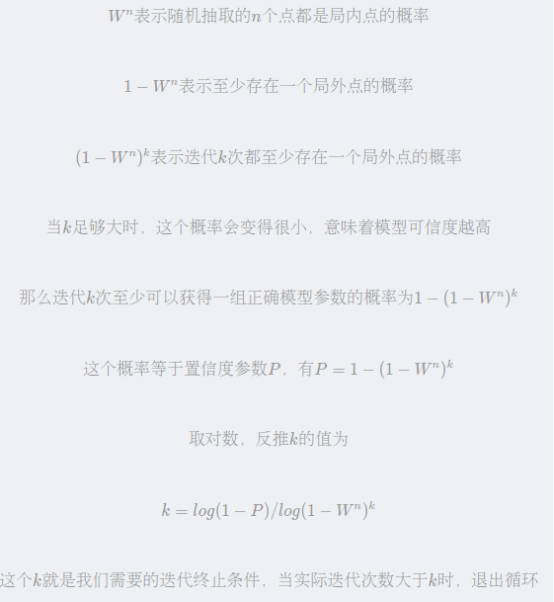代码如下：
#include <iostream>
#include <pcl/io/io.h>
#include <pcl/io/pcd_io.h>
#include <pcl/point_types.h>
#include <pcl/visualization/cloud_viewer.h>
#include <unordered_set>

using namespace std;
int main()
{
pcl::PointCloud<pcl::PointXYZ>::Ptr cloud(new pcl::PointCloud<pcl::PointXYZ>);
pcl::PCDReader reader;
reader.read("D:\\SFND_Lidar_Obstacle_Detection\\SFND_Lidar_Obstacle_Detection代码分解\\cloudRegion.pcd", *cloud);

int num_points = cloud->points.size();
//cout << num_points << endl; //2063
std::unordered_set<int> inliersResult;

int maxIterations = 40; //迭代次数
while (maxIterations--)  //
{
std::unordered_set<int> inliers;  //存放平面的内点，平面上的点也属于平面的内点
//因为一开始定义inliers，内点并没有存在，所以随机在原始点云中随机选取了三个点作为点云的求取平面系数的三个点
while (inliers.size() < 3)  //当内点小于3 就随机选取一个点 放入内点中 也就是需要利用到三个内点
{
inliers.insert(rand() % num_points);   //产生 0~num_points 中的一个随机数
}

// 需要至少三个点 才能找到地面
float x1, y1, z1, x2, y2, z2, x3, y3, z3;
auto itr = inliers.begin();  //auto 自动类型
x1 = cloud->points[*itr].x;
y1 = cloud->points[*itr].y;
z1 = cloud->points[*itr].z;
itr++;
x2 = cloud->points[*itr].x;
y2 = cloud->points[*itr].y;
z2 = cloud->points[*itr].z;
itr++;
x3 = cloud->points[*itr].x;
y3 = cloud->points[*itr].y;
z3 = cloud->points[*itr].z;

//计算平面系数
float a, b, c, d, sqrt_abc;
a = (y2 - y1) * (z3 - z1) - (z2 - z1) * (y3 - y1);
b = (z2 - z1) * (x3 - x1) - (x2 - x1) * (z3 - z1);
c = (x2 - x1) * (y3 - y1) - (y2 - y1) * (x3 - x1);
d = -(a * x1 + b * y1 + c * z1);
sqrt_abc = sqrt(a * a + b * b + c * c);

//分别计算这些点到平面的距离
for (int i = 0; i < num_points; i++)
{
if (inliers.count(i) > 0) //判断一下有没有内点
{ // that means: if the inlier in already exist, we dont need do anymore
continue;
}
pcl::PointXYZ point = cloud->points[i];
float x = point.x;
float y = point.y;
float z = point.z;
float dist = fabs(a * x + b * y + c * z + d) / sqrt_abc; // calculate the distance between other points and plane
float distanceTol = 0.3;
if (dist < distanceTol)
{
inliers.insert(i); //如果点云中的点 距离平面距离的点比较远 那么该点则视为内点
}
//将inliersResult 中的内容不断更新，因为地面的点一定是最多的，所以迭代40次 找到的inliersResult最大时，也就相当于找到了地面
//inliersResult 中存储的也就是地面上的点云
if (inliers.size() > inliersResult.size())
{
inliersResult = inliers;
}
}
//cout << inliers.size() << endl;
}
//迭代结束后，所有属于平面上的内点都存放在inliersResult中
//std::unordered_set<int> inliersResult;
cout << inliersResult.size() << endl;  //1633

//创建两片点云，一片存放地面，一片存放其他点
pcl::PointCloud<pcl::PointXYZ>::Ptr out_plane(new pcl::PointCloud<pcl::PointXYZ>);
pcl::PointCloud<pcl::PointXYZ>::Ptr in_plane(new pcl::PointCloud<pcl::PointXYZ>);

for (int i = 0; i < num_points; i++)
{
pcl::PointXYZ pt = cloud->points[i];
if (inliersResult.count(i))
{
out_plane->points.push_back(pt);
}
else
{
in_plane->points.push_back(pt);
}
}

/*pcl::PCDWriter writer;
writer.write("C:\\Users\\Administrator\\Downloads\\求助\\求助\\tree-2-Rend.pcd", *cloud_filtered1);*/
pcl::visualization::PCLVisualizer::Ptr viewer(new pcl::visualization::PCLVisualizer("显示窗口"));  //窗口显示点云
viewer->addPointCloud(in_plane, "*cloud");
viewer->resetCamera();		//相机点重置
viewer->spin();
system("pause");
return (0);
}

参考:
https://blog.csdn.net/dengxiong_bright/article/details/102725848
https://blog.csdn.net/qinlele1994/article/details/106287529


展开全文• 为了解决这些问题，本文将介绍基于随机采样的路径规划算法。这类算法适用于高维度空间，它们以概率完备性(当时间接近无限时一定有解)来代替完备性，从而提高搜索效率。 概率路图算法(Probabilistic Road Map, PRM) ...
基于图搜索的路径规划算法主要用于低维度空间上的路径规划问题，它在这类问题中往往具有较好的完备性，但是需要对环境进行完整的建模工作，在高维度空间中往往会出现维数灾难。为了解决这些问题，本文将介绍基于随机采样的路径规划算法。这类算法适用于高维度空间，它们以概率完备性(当时间接近无限时一定有解)来代替完备性，从而提高搜索效率。
基于随机采样的路径规划算法又分为单查询算法(single-query path planning)以及渐近最优算法(asymptotically optimal path planning)，前者只要找到可行路径即可，侧重快速性，后者还会对找到的路径进行逐步优化，慢慢达到最优，侧重最优性。本文介绍的单查询方法为概率路图算法(Probabilistic Road Map, PRM)、快速随机扩展树算法(Rapidly-exploring Random Tree, RRT)、RRT-Connect算法，渐近最优算法有RRT*算法。
概率路图算法(Probabilistic Road Map, PRM)
PRM算法首先使用随机采样的方式在环境中建立路径网络图，将连续的空间转换为离散的空间，然后在路径网络图上进行路径规划，解决在高维空间中搜索效率低的问题。
算法流程如下：

采样：在地图中随机撒点，剔除落在障碍物上的点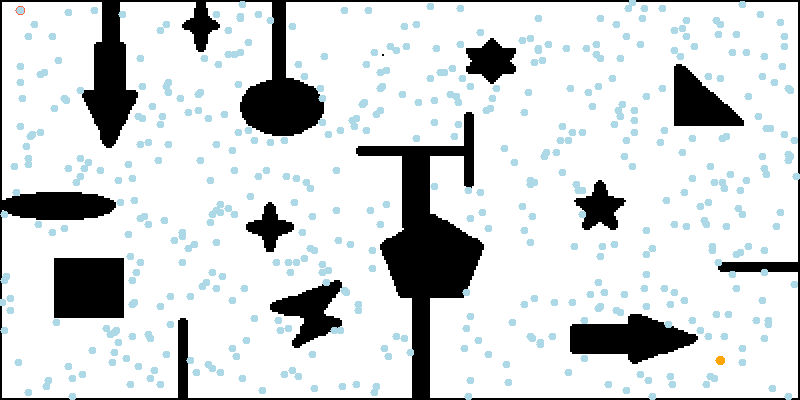生成概率路图：根据点与点间的距离和是否存在直线通路将上步中得到的采样点进行连接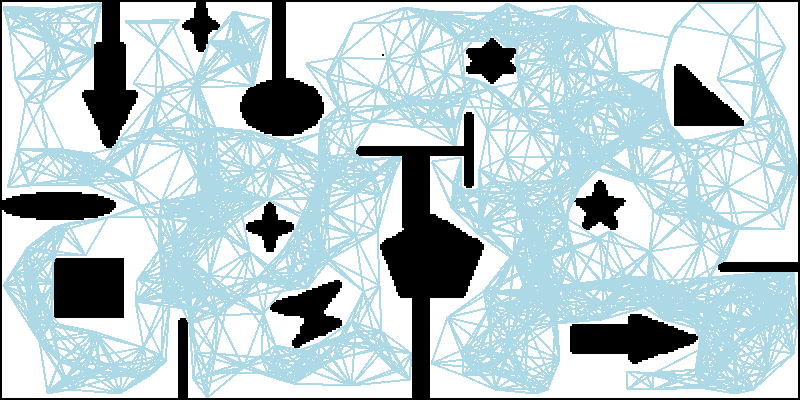搜索路径：使用图搜索算法(如Dijkstra算法)在上步得到的路图中搜索出一条从起点到终点的最短路径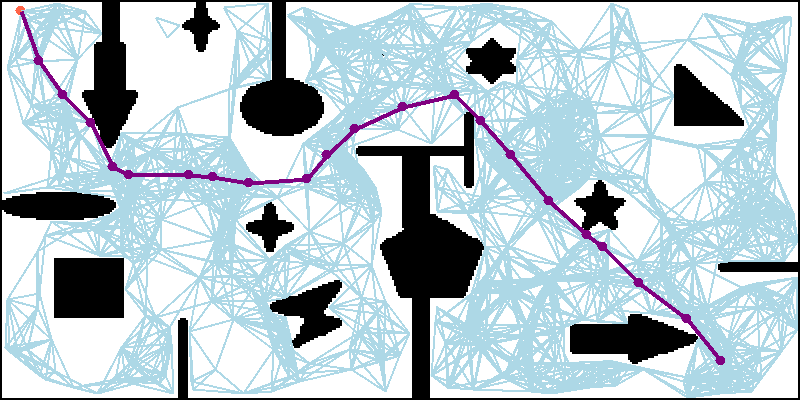其中采样点的数量和采样点间存在通路的最大距离是路径规划成功与否的关键。
采样点太少，可能会导致路径规划失败，下图(a)中不能生成完整的路图，导致规划失败；而通样是300个采样点，图(b)则能生成一个完整的路图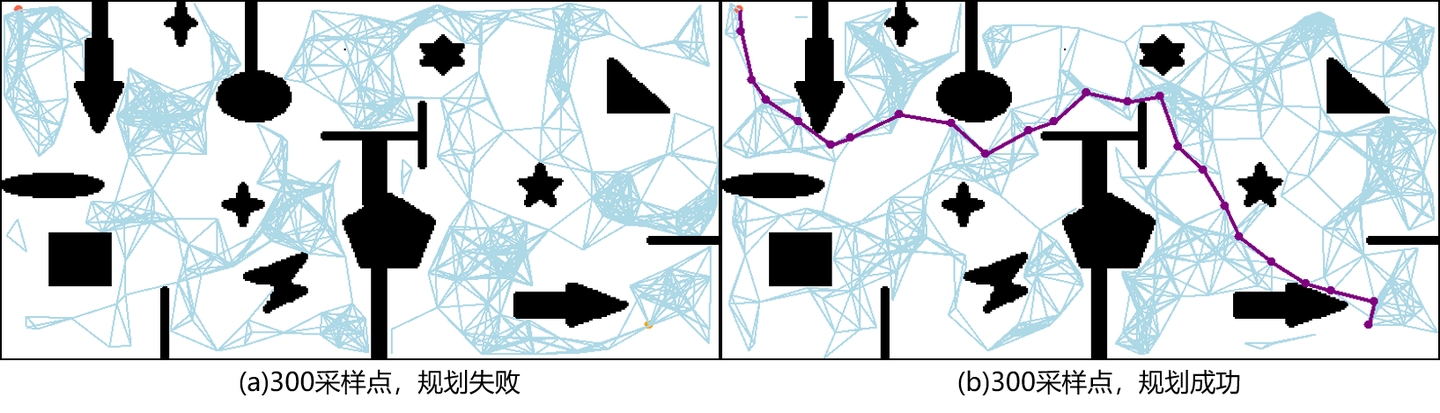采样点数量增加，搜索到的路径会逐渐接近最短路径，但同时搜索效率会降低，如下图：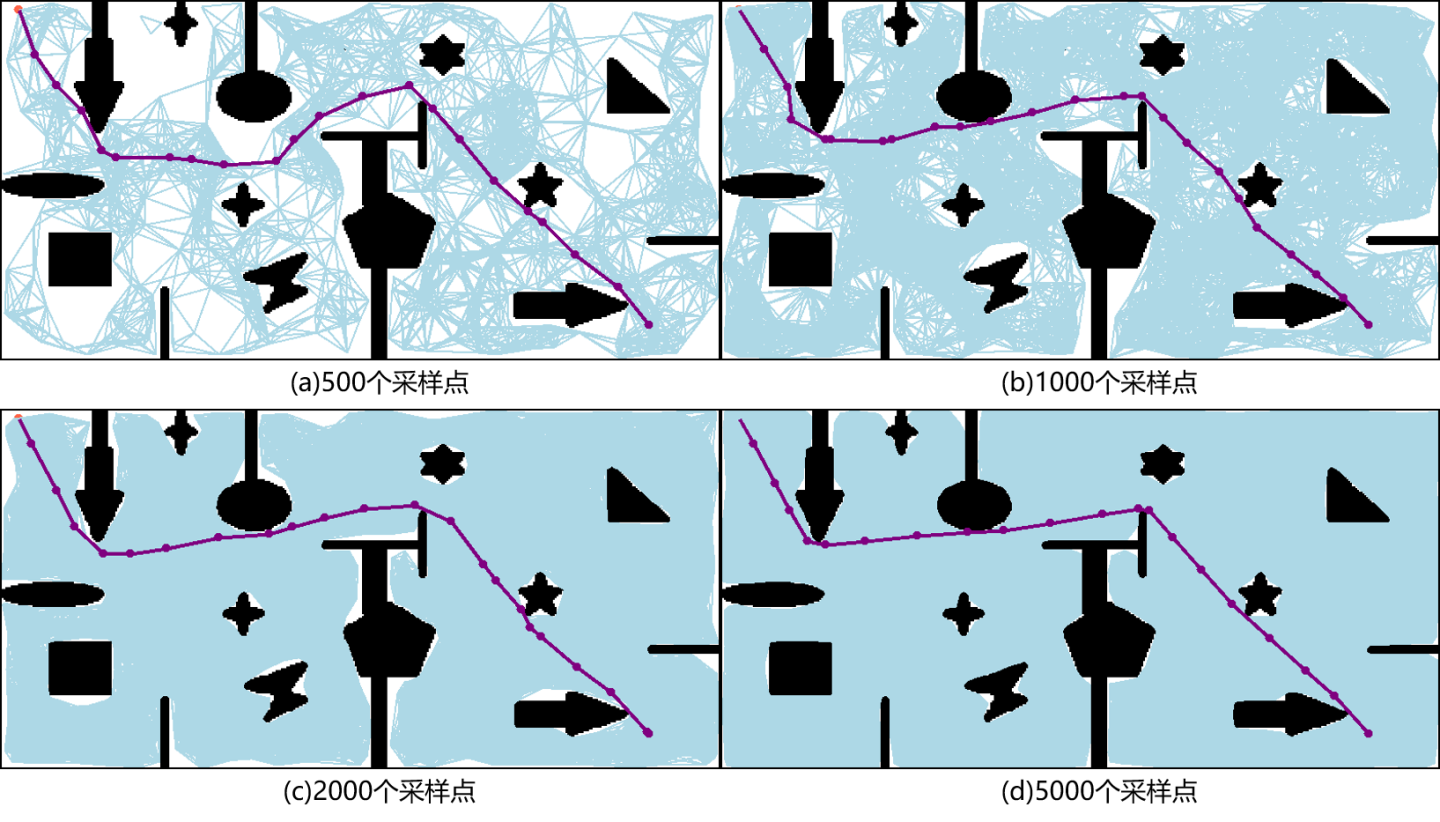采样点间存在通路的最大距离对规划结果的影响和以上类似：距离太小，会导致规划失败；距离太大，会降低搜索效率。如下图(a)，由于设置的最大距离太小，不能生成一张完整的路图，导致规划失败；而图(b)虽然能找到路径，但是生成的路图中存在很多冗余通路。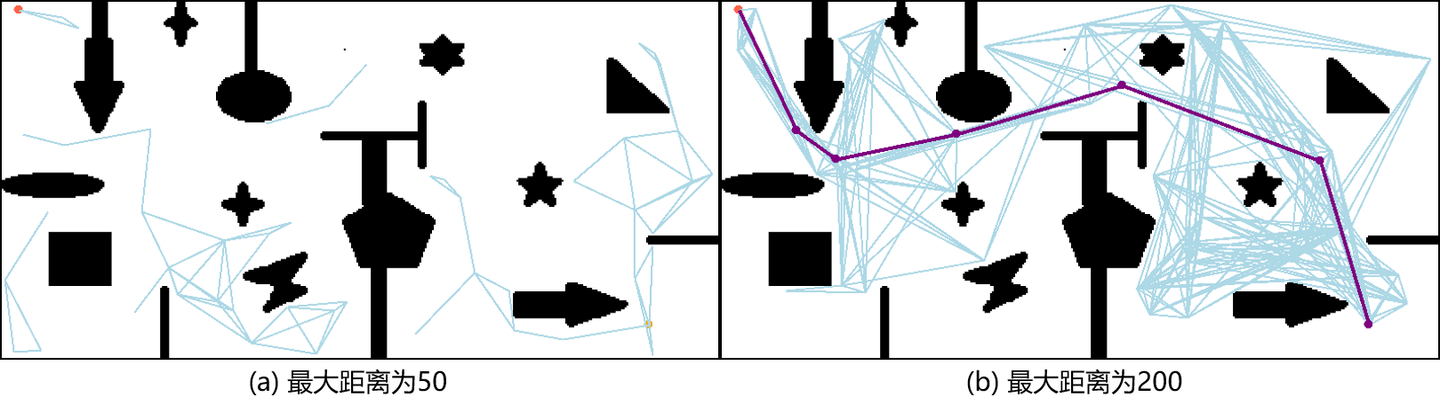PRM算法参数少、结构简单，能够提高高维空间搜索效率，也能在生成概率路图时添加机器人的运动学约束，使最终生成的路径符合机器人的运动学模型。同时，随机采样得到的概率路图只需要建立一次就可以一直使用，重用性强。但由于采样过程是完全随机的，得到的节点大多数都偏离最终路径，会增加额外的计算量。
快速随机扩展树算法(Rapidly-exploring Random Tree, RRT)
RRT算法是一种单查询(single-query)算法，目标是尽可能快的找到一条从起点到终点的可行路径。它的搜索过程类似于一棵树不断生长、向四周扩散的过程，它以起点作为根节点构建一棵搜索树$T$。它的算法流程用伪代码描述如下：

$T.init(x_{init})$
$for$ $i = 1, ..., n$ $do$
​	$x_{rand}←SampleFree_i$;
​	$x_{near}←Nearest(T, x_{rand})$;
​	$x_{new}←Steer(x_{near}, x_{rand})$;
​	$if$ $ObtacleFree(X_{near}, x_{new})$ $then$
​		$T.add\_vertex(x_{new})$
​		$T.add\_edge(x_{near}, x_{new})$
​		$V←V\cup\{x_{new}\}; E←E\cup\{x_{near}, x_{new}\}$;
$return$ $G=(V, E)$;

其中$x_{init}$表示起点，$T$表示随机树，在算法开始时，将起点$x_{init}$加入到随机树。接下来使用$SampleFree$函数在自由空间中随机采样，获得一个采样点$x_{rand}$，再使用$Nearest$函数获得随机树中距离$x_{rand}$最近的一个节点$x_{near}$；使用$Steer$函数在$x_{near}$和$x_{rand}$的连线上距离$x_{near}$步长$u$的位置生成一个节点$x_{new}$，使用$ObtacleFree$函数判断$x_{near}$和$x_{new}$间是否存在直线通路，若存在则将$x_{new}$加入到随机树$T$的节点集合中，同时将$x_{nearest}$作为$x_{new}$的父节点，将边$(x_{nearest}, x_{new})$加入到随机树$T$的边集中
扩展流程如图：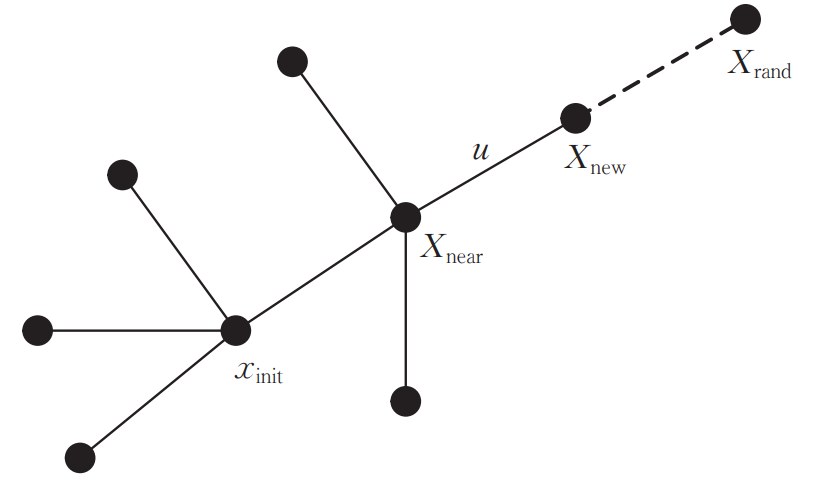上述是基础的RRT算法流程，它的采样过程是完全随机的，还可以在采样时以一定的概率直接采样终点作为$x_{rand}$，加快搜索速度。
RRT作为一种随机采样的规划算法，它的复杂度也不受地图的离散程度影响，在高维空间中仍具有很高的搜索效率。但是前面提到RRT是一种单查询算法，只管尽快地找到可行路径，所以最终路径并不是最优的，甚至会非常“绕”。
RRT-Connect
RRT-Connect在RRT的基础上引入了双树扩展环节，分别以起点和目标点为根节点生成两棵树进行双向扩展，当两棵树建立连接时可认为路径规划成功。通过一次采样得到一个采样点$x_{rand}$，然后两棵搜索树同时向采样点$x_{rand}$方向进行扩展，加快两棵树建立连接的速度。相较于单树扩展的RRT算法，RRT-Connect加入了启发式步骤，加快了搜索速度，对于狭窄通道也具有较好的效果。但是RRT-Connect和RRT一样，都是单查询算法，最终路径并不是最优的。接下来介绍基于随机采样的渐近最优路径规划算法。
RRT*
RRT*算法是一种渐近最优算法。算法流程与RRT算法流程基本相同，不同之处就在于最后加入将$X_{new}$加入搜索树$T$时父节点的选择策略。
RRT*算法在选择父节点时会有一个**重连(Rewire)**过程，也就是在以$X_{new}$为圆心、半径为$r$的邻域内，找到与$X_{new}$连接后路径代价(从起点移动到$X_{new}$的路径长度)最小的节点$X_{min}$，并重新选择$X_{min}$作为$X_{new}$的父节点，而不是$X_{near}$。重连过程的示意图如下：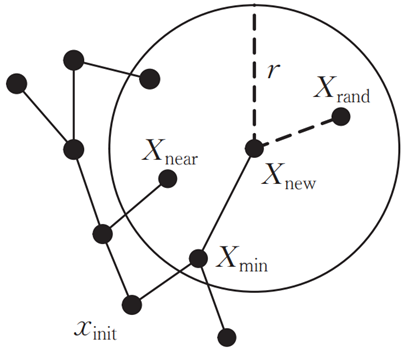参考
Lavalle S M . Rapidly-Exploring Random Trees: A New Tool for Path Planning[J]. Research Report, 1999.
Jr J ,  Lavalle S M . RRT-Connect: An Efficient Approach to Single-Query Path Planning[C]// Proceedings of the 2000 IEEE International Conference on Robotics and Automation, ICRA 2000, April 24-28, 2000, San Francisco, CA, USA. IEEE, 2000.
Karaman S , Frazzoli E . Sampling-based Algorithms for Optimal Motion Planning[J]. The International Journal of Robotics Research, 2011, 30(7):846-894.


展开全文• #include <iostream> #include <algorithm> #include <iterator> #include <vector> //假设这n个数的序号依次为0,1,2,...,n-1,数组名为num void knuth1(std::vector<... i.
• //存放平面的内点，平面上的点也属于平面的内点 //因为一开始定义inliers，内点并没有存在，所以随机在原始点云中随机选取了三个点作为点云的求取平面系数的三个点 while (inliers.size() ) //当内点小于3 就随机...
• // 随机填充点云 cloud_in->width = 300; //设置点云宽度 cloud_in->height = 1; //设置点云为无序点 cloud_in->is_dense = false; cloud_in->points.resize(cloud_in->width * cloud_in->height); for (size_t...
• 基于稀疏相似网络,提出了一种改进后的随机聚类采样算法对网络社团进行探测。在人工和真实网络上,将算法与未改进的随机聚类采样算法以及几种典型的社团探测算法进行了准确率和时间复杂度的比较。实验结果表明,该方法...
• 这篇文章系统详尽地介绍了经典的随机采样一致算法RANSAC，希望能对大家有所帮助
• RANSAC(RAndomSAmpleConsensus,随机采样一致)算法是从一组含有“外点”(outliers)的数据中正确估计数学模型参数的迭代算法。“外点”一般指的的数据中的噪声，比如说匹配中的误匹配和估计曲线中的离群点。所以，...
• 为解决多标签学习中数据不平衡、传统重采样过程标签样本集相互影响以及弱势类信息大量重复和强势类信息大量丢失的问题，提出多标签随机均衡采样算法。该算法在多标签的条件下提出随机均衡采样思想，充分利用强势类和...多标签学习 数据不平衡
• 对于随机采样算法，在采样不全时，通常不能保证找到最优解，只能尽量找。根据搜索的方式，可以把随机算法分成两类： 蒙特卡罗方法（Monte Carlo Simulation）：采样越多，越近似最优解； 拉斯维加斯方法（Las Vegas...
• 基于稀疏相似网络, 提出了一种改进后的随机聚类采样算法对网络社团进行探测。在人工和真实网络上, 将算法与未改进的随机聚类采样算法以及几种典型的社团探测算法进行了准确率和时间复杂度的比较。实验结果表明, 该...复杂网络 相似性
• 这些将分别称为加权和非加权随机采样算法。 在非加权算法中，流中的每个项目出现在样本中的概率为k/n 。 在加权算法中，这种可能性取决于额外的参数weight 。 每种算法可能以不同的方式解释此参数，例如，在中...
• 最近在学习点云的分割算法，目前了解到有两种分割算法，一种是聚类分割算法，一种是随机采样一致性算法，聚类算法上篇文章已经有所提及。今天分析下随机采样一致性算法，然后代码理解； RANSAC随机采样一致性算法...
• RANSAC随机采样一致性算法RANSAC算法的输入是一组观测数据（往往含有较大的噪声或无效点），它是一种重采样技术（resampling technique），通过估计模型参数所需的最小的样本点数，来得到备选模型集合，然后在不断的...
• 随机采样一致性算法在计算机视觉领域有着广泛的运用，在点云分割中，经常用到随机采样一致性算法，所以就仔细研究了一下该算法，并记下了一些便于理解的笔记。 采样一致性参数估计算法主要用于排除错误样本，可以从...
• Select four feature pairs (at random) 随机找4对特征点 Compute homography H 计算H Compute inliers where ||pi’, H pi|| ε (if not enough times, goto 1.) 找到符合H的inliers Keep largest set of ...
• 学习链接在学习LDA算法原理的时候，我们是用吉布斯采样来学习参数，吉布斯采样是随机采样算法的一种。
•研究论文
• RANSAC 随机采样一致性算法 RANSAC是一种随机参数估计算法。RANSAC从样本中随机抽选出一个样本子集，使用最小方差估计算法对这个子集计算模型参数，然后计算所有样本与该模型的偏差，在使用一个预先设定好的阈值与...
• 点击上方“3D视觉工坊”，选择“星标”干货第一时间送达Summary为解决2D和3D图像数据中噪声多的问题，本文将简述RANSAC算法是如何鲁棒地估计模型参数，从包含噪声点的数据集中估计出...人工智能 机器学习 深度学习 计算机视觉
• 鉴于深度学习在学术界和工业界的重要性，向对目前有代表性的深度学习数据随机采样算法进行归纳和总结，综述了不同类型随机采样算法的基本思想和方法。首先介绍了深度学习和随机采样的概念；然后根据深度学习算法的...深度学习
• 在计算机视觉领域广泛的使用各种不同的采样一致性参数估计算法用于排除错误的样本，样本不同对应的应用不同，例如剔除错误的配准点对，分割出处在模型上的点集，PCL中以随机采样一致性算法（RANSAC）为核心，同时...三维重建
• 算法简介：从样本中抽取一个子集，通过该算法最小方差对这个样本计算出这个该模型的参数（例如模型是一条直线，那么计算该样本所有的点到直线的方差，综合最短的距离就是这条直线的位置），然后所有样本与该模型的...c++...